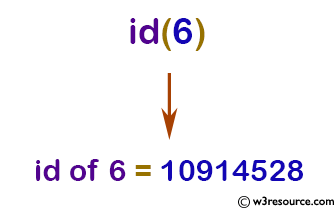# Python: id() function

## id()

The id() function is used to get the identity of an object.

The identity is a unique integer for that object during its lifetime. This is also the address of the object in memory.

Version:

(Python 3.2.5)

Syntax:

```id(object)
```

Parameter:

Name Description Required /
Optional
object An object, int, float, str,list, dict, tuple etc. Required

Example: Python id() function

``````print('id of 6 =',id(6))

x = 5
print('id of x =',id(x))

y = x
print('id of y =',id(y))

z = 5.0
print('id of z =',id(z))
``````

Output:

```id of 6 = 10914528
id of x = 10914496
id of y = 10914496
id of z = 140486394610168
```

Pictorial Presentation:Python Code Editor:

Previous: hex()
Next: input()

Test your Python skills with w3resource's quiz

﻿

## Python: Tips of the Day

How do I check if a list is empty?

For example, if passed the following:

```a = []
if not a:
print("List is empty")
```

Ref: https://bit.ly/2A4JXx9